Anúncio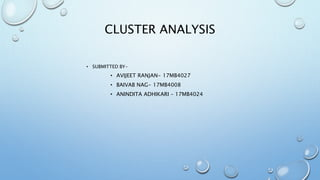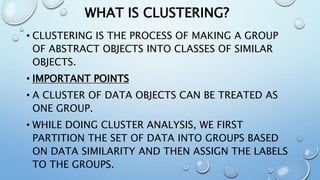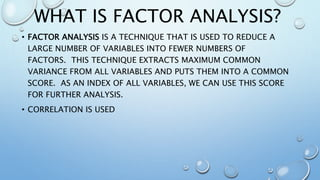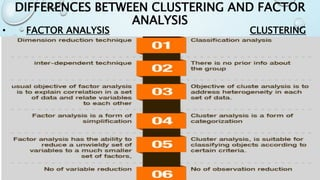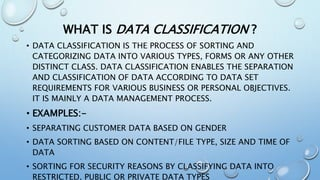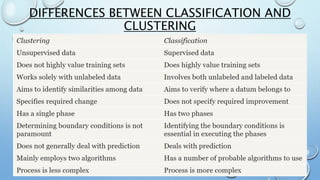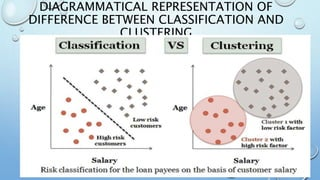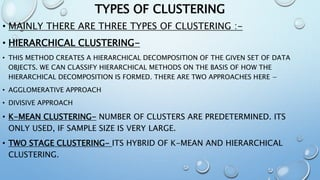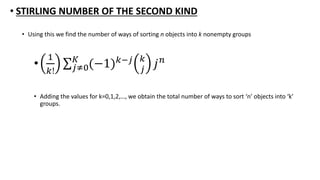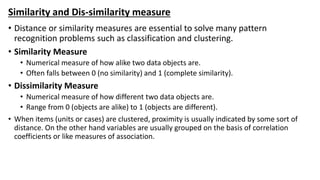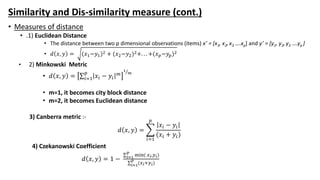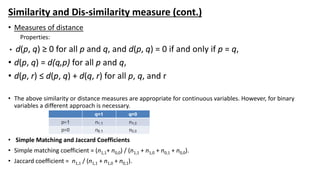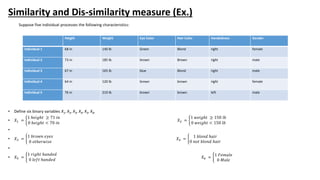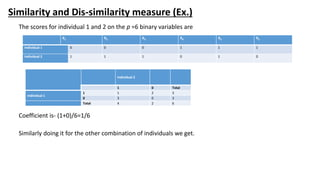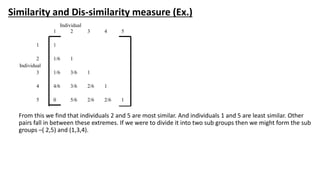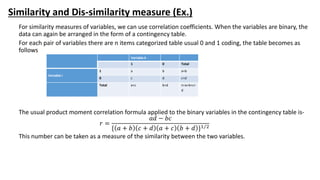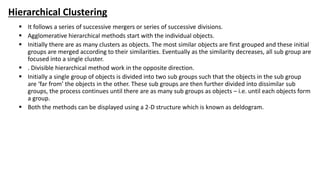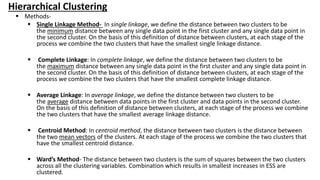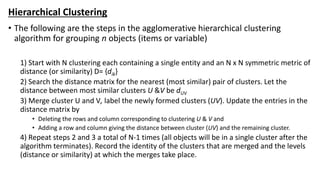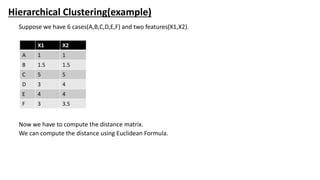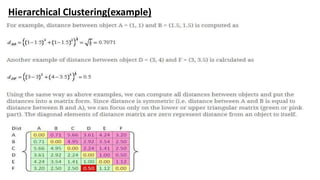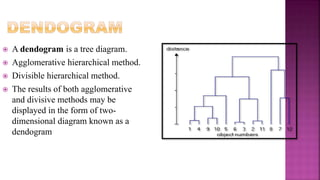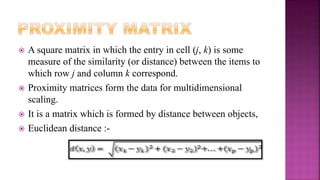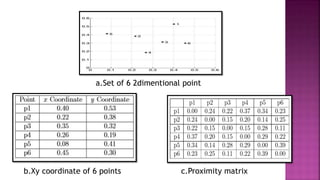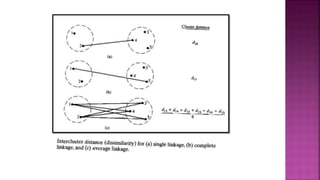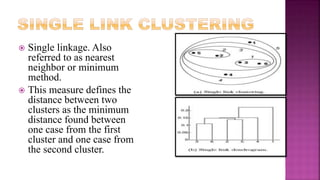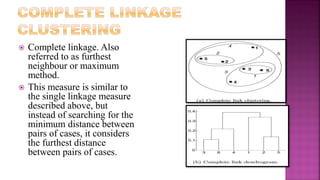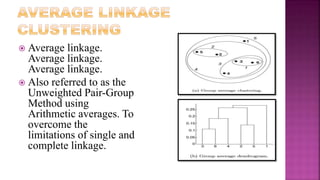1 de 28
Anúncio

### Cluster Analysis

1. CLUSTER ANALYSIS • SUBMITTED BY- • AVIJEET RANJAN- 17MB4027 • BAIVAB NAG- 17MB4008 • ANINDITA ADHIKARI – 17MB4024
2. WHAT IS CLUSTERING? • CLUSTERING IS THE PROCESS OF MAKING A GROUP OF ABSTRACT OBJECTS INTO CLASSES OF SIMILAR OBJECTS. • IMPORTANT POINTS • A CLUSTER OF DATA OBJECTS CAN BE TREATED AS ONE GROUP. • WHILE DOING CLUSTER ANALYSIS, WE FIRST PARTITION THE SET OF DATA INTO GROUPS BASED ON DATA SIMILARITY AND THEN ASSIGN THE LABELS TO THE GROUPS.
3. WHAT IS FACTOR ANALYSIS? • FACTOR ANALYSIS IS A TECHNIQUE THAT IS USED TO REDUCE A LARGE NUMBER OF VARIABLES INTO FEWER NUMBERS OF FACTORS. THIS TECHNIQUE EXTRACTS MAXIMUM COMMON VARIANCE FROM ALL VARIABLES AND PUTS THEM INTO A COMMON SCORE. AS AN INDEX OF ALL VARIABLES, WE CAN USE THIS SCORE FOR FURTHER ANALYSIS. • CORRELATION IS USED
4. DIFFERENCES BETWEEN CLUSTERING AND FACTOR ANALYSIS • FACTOR ANALYSIS CLUSTERING
5. WHAT IS DATA CLASSIFICATION ? • DATA CLASSIFICATION IS THE PROCESS OF SORTING AND CATEGORIZING DATA INTO VARIOUS TYPES, FORMS OR ANY OTHER DISTINCT CLASS. DATA CLASSIFICATION ENABLES THE SEPARATION AND CLASSIFICATION OF DATA ACCORDING TO DATA SET REQUIREMENTS FOR VARIOUS BUSINESS OR PERSONAL OBJECTIVES. IT IS MAINLY A DATA MANAGEMENT PROCESS. • EXAMPLES:- • SEPARATING CUSTOMER DATA BASED ON GENDER • DATA SORTING BASED ON CONTENT/FILE TYPE, SIZE AND TIME OF DATA • SORTING FOR SECURITY REASONS BY CLASSIFYING DATA INTO RESTRICTED, PUBLIC OR PRIVATE DATA TYPES
6. DIFFERENCES BETWEEN CLASSIFICATION AND CLUSTERING
7. DIAGRAMMATICAL REPRESENTATION OF DIFFERENCE BETWEEN CLASSIFICATION AND CLUSTERING
8. TYPES OF CLUSTERING • MAINLY THERE ARE THREE TYPES OF CLUSTERING :- • HIERARCHICAL CLUSTERING- • THIS METHOD CREATES A HIERARCHICAL DECOMPOSITION OF THE GIVEN SET OF DATA OBJECTS. WE CAN CLASSIFY HIERARCHICAL METHODS ON THE BASIS OF HOW THE HIERARCHICAL DECOMPOSITION IS FORMED. THERE ARE TWO APPROACHES HERE − • AGGLOMERATIVE APPROACH • DIVISIVE APPROACH • K-MEAN CLUSTERING- NUMBER OF CLUSTERS ARE PREDETERMINED. ITS ONLY USED, IF SAMPLE SIZE IS VERY LARGE. • TWO STAGE CLUSTERING- ITS HYBRID OF K-MEAN AND HIERARCHICAL CLUSTERING.
9. • STIRLING NUMBER OF THE SECOND KIND • Using this we find the number of ways of sorting n objects into k nonempty groups • 1 𝑘! 𝑗≠0 𝐾 (−1) 𝑘−𝑗 𝑘 𝑗 𝑗 𝑛 • Adding the values for k=0,1,2,…, we obtain the total number of ways to sort ‘n’ objects into ‘k’ groups.
10. Similarity and Dis-similarity measure • Distance or similarity measures are essential to solve many pattern recognition problems such as classification and clustering. • Similarity Measure • Numerical measure of how alike two data objects are. • Often falls between 0 (no similarity) and 1 (complete similarity). • Dissimilarity Measure • Numerical measure of how different two data objects are. • Range from 0 (objects are alike) to 1 (objects are different). • When items (units or cases) are clustered, proximity is usually indicated by some sort of distance. On the other hand variables are usually grouped on the basis of correlation coefficients or like measures of association.
11. Similarity and Dis-similarity measure (cont.) • Measures of distance • .1) Euclidean Distance • The distance between two p dimensional observations (items) x’ = [x1, x2,x3 ….xp] and y’ = [y1, y2,y3 ….yp ] • 𝑑 𝑥, 𝑦 = (𝑥1−𝑦1)2 + (𝑥2−𝑦2)2+. . . +(𝑥 𝑝−𝑦𝑝)2 • 2) Minkowski Metric • 𝑑 𝑥, 𝑦 = 𝑖=1 𝑝 𝑥𝑖 − 𝑦𝑖 𝑚 1 𝑚 • m=1, it becomes city block distance • m=2, it becomes Euclidean distance 3) Canberra metric :- 𝑑 𝑥, 𝑦 = 𝑖=1 𝑝 𝑥𝑖 − 𝑦𝑖 (𝑥𝑖 + 𝑦𝑖) 4) Czekanowski Coefficient 𝑑 𝑥, 𝑦 = 1 − 𝑖=1 𝑝 min( 𝑥 𝑖,𝑦 𝑖) 𝑖=1 𝑝 (𝑥 𝑖+𝑦 𝑖)
12. Similarity and Dis-similarity measure (cont.) • Measures of distance Properties: • d(p, q) ≥ 0 for all p and q, and d(p, q) = 0 if and only if p = q, • d(p, q) = d(q,p) for all p and q, • d(p, r) ≤ d(p, q) + d(q, r) for all p, q, and r • The above similarity or distance measures are appropriate for continuous variables. However, for binary variables a different approach is necessary. • Simple Matching and Jaccard Coefficients • Simple matching coefficient = (n1,1+ n0,0) / (n1,1 + n1,0 + n0,1 + n0,0). • Jaccard coefficient = n1,1 / (n1,1 + n1,0 + n0,1).
13. Similarity and Dis-similarity measure (Ex.) Suppose five individual processes the following characteristics: • Define six binary variables X1, X2, X3, X4, X5, X6, • 𝑋1 = 1 ℎ𝑒𝑖𝑔ℎ𝑡 ≥ 71 𝑖𝑛 0 ℎ𝑒𝑖𝑔ℎ𝑡 < 70 𝑖𝑛 𝑋2 = 1 𝑤𝑒𝑖𝑔ℎ𝑡 ≥ 150 𝑙𝑏 0 𝑤𝑒𝑖𝑔ℎ𝑡 < 150 𝑙𝑏 • • 𝑋3 = 1 𝑏𝑟𝑜𝑤𝑛 𝑒𝑦𝑒𝑠 0 𝑜𝑡ℎ𝑒𝑟𝑤𝑖𝑠𝑒 𝑋4 = 1 𝑏𝑙𝑜𝑛𝑑 ℎ𝑎𝑖𝑟 0 𝑛𝑜𝑡 𝑏𝑙𝑜𝑛𝑑 ℎ𝑎𝑖𝑟 • • 𝑋5 = 1 𝑟𝑖𝑔ℎ𝑡 ℎ𝑎𝑛𝑑𝑒𝑑 0 𝑙𝑒𝑓𝑡 ℎ𝑎𝑛𝑑𝑒𝑑 𝑋6 = 1 𝐹𝑒𝑚𝑎𝑙𝑒 0 𝑀𝑎𝑙𝑒 Height Weight Eye Color Hair Color Handedness Gender Individual 1 68 in 140 lb Green Blond right female Individual 2 73 in 185 lb brown Brown right male Individual 3 67 in 165 lb blue Blond right male Individual 4 64 in 120 lb brown brown right female Individual 5 76 in 210 lb brown brown left male
14. Similarity and Dis-similarity measure (Ex.) The scores for individual 1 and 2 on the p =6 binary variables are Coefficient is- (1+0)/6=1/6 Similarly doing it for the other combination of individuals we get. X1 X2 X3 X4 X5 X5 Individual 1 0 0 0 1 1 1 Individual 2 1 1 1 0 1 0 Individual 2 1 0 Total Individual 1 1 1 2 3 0 3 0 3 Total 4 2 6
15. Similarity and Dis-similarity measure (Ex.) From this we find that individuals 2 and 5 are most similar. And individuals 1 and 5 are least similar. Other pairs fall in between these extremes. If we were to divide it into two sub groups then we might form the sub groups –( 2,5) and (1,3,4). Individual 1 2 3 4 5 1 1 2 1/6 1 Individual 3 1/6 3/6 1 4 4/6 3/6 2/6 1 5 0 5/6 2/6 2/6 1
16. Similarity and Dis-similarity measure (Ex.) For similarity measures of variables, we can use correlation coefficients. When the variables are binary, the data can again be arranged in the form of a contingency table. For each pair of variables there are n items categorized table usual 0 and 1 coding, the table becomes as follows The usual product moment correlation formula applied to the binary variables in the contingency table is- 𝑟 = 𝑎𝑑 − 𝑏𝑐 { 𝑎 + 𝑏 𝑐 + 𝑑 𝑎 + 𝑐 𝑏 + 𝑑 }1 2 This number can be taken as a measure of the similarity between the two variables. Variable k 1 0 Total Variable i 1 a b a+b 0 c d c+d Total a+c b+d n=a+b+c+ d
17. Hierarchical Clustering  It follows a series of successive mergers or series of successive divisions.  Agglomerative hierarchical methods start with the individual objects.  Initially there are as many clusters as objects. The most similar objects are first grouped and these initial groups are merged according to their similarities. Eventually as the similarity decreases, all sub group are focused into a single cluster.  . Divisible hierarchical method work in the opposite direction.  Initially a single group of objects is divided into two sub groups such that the objects in the sub group are ‘far from’ the objects in the other. These sub groups are then further divided into dissimilar sub groups, the process continues until there are as many sub groups as objects – i.e. until each objects form a group.  Both the methods can be displayed using a 2-D structure which is known as deldogram.
18. Hierarchical Clustering  Methods-  Single Linkage Method- In single linkage, we define the distance between two clusters to be the minimum distance between any single data point in the first cluster and any single data point in the second cluster. On the basis of this definition of distance between clusters, at each stage of the process we combine the two clusters that have the smallest single linkage distance.  Complete Linkage: In complete linkage, we define the distance between two clusters to be the maximum distance between any single data point in the first cluster and any single data point in the second cluster. On the basis of this definition of distance between clusters, at each stage of the process we combine the two clusters that have the smallest complete linkage distance.  Average Linkage: In average linkage, we define the distance between two clusters to be the average distance between data points in the first cluster and data points in the second cluster. On the basis of this definition of distance between clusters, at each stage of the process we combine the two clusters that have the smallest average linkage distance.  Centroid Method: In centroid method, the distance between two clusters is the distance between the two mean vectors of the clusters. At each stage of the process we combine the two clusters that have the smallest centroid distance.  Ward’s Method- The distance between two clusters is the sum of squares between the two clusters across all the clustering variables. Combination which results in smallest increases in ESS are clustered.
19. Hierarchical Clustering • The following are the steps in the agglomerative hierarchical clustering algorithm for grouping n objects (items or variable) 1) Start with N clustering each containing a single entity and an N x N symmetric metric of distance (or similarity) D= {dik} 2) Search the distance matrix for the nearest (most similar) pair of clusters. Let the distance between most similar clusters U &V be dUV 3) Merge cluster U and V, label the newly formed clusters (UV). Update the entries in the distance matrix by • Deleting the rows and column corresponding to clustering U & V and • Adding a row and column giving the distance between cluster (UV) and the remaining cluster. 4) Repeat steps 2 and 3 a total of N-1 times (all objects will be in a single cluster after the algorithm terminates). Record the identity of the clusters that are merged and the levels (distance or similarity) at which the merges take place.
20. Hierarchical Clustering(example) Suppose we have 6 cases(A,B,C,D,E,F) and two features(X1,X2). Now we have to compute the distance matrix. We can compute the distance using Euclidean Formula. X1 X2 A 1 1 B 1.5 1.5 C 5 5 D 3 4 E 4 4 F 3 3.5
21. Hierarchical Clustering(example)
22.  A dendogram is a tree diagram.  Agglomerative hierarchical method.  Divisible hierarchical method.  The results of both agglomerative and divisive methods may be displayed in the form of two- dimensional diagram known as a dendogram
23.  A square matrix in which the entry in cell (j, k) is some measure of the similarity (or distance) between the items to which row j and column k correspond.  Proximity matrices form the data for multidimensional scaling.  It is a matrix which is formed by distance between objects,  Euclidean distance :-
24. a.Set of 6 2dimentional point b.Xy coordinate of 6 points c.Proximity matrix
25.  Single linkage. Also referred to as nearest neighbor or minimum method.  This measure defines the distance between two clusters as the minimum distance found between one case from the first cluster and one case from the second cluster.
26.  Complete linkage. Also referred to as furthest neighbour or maximum method.  This measure is similar to the single linkage measure described above, but instead of searching for the minimum distance between pairs of cases, it considers the furthest distance between pairs of cases.
27.  Average linkage. Average linkage. Average linkage.  Also referred to as the Unweighted Pair-Group Method using Arithmetic averages. To overcome the limitations of single and complete linkage.
Anúncio